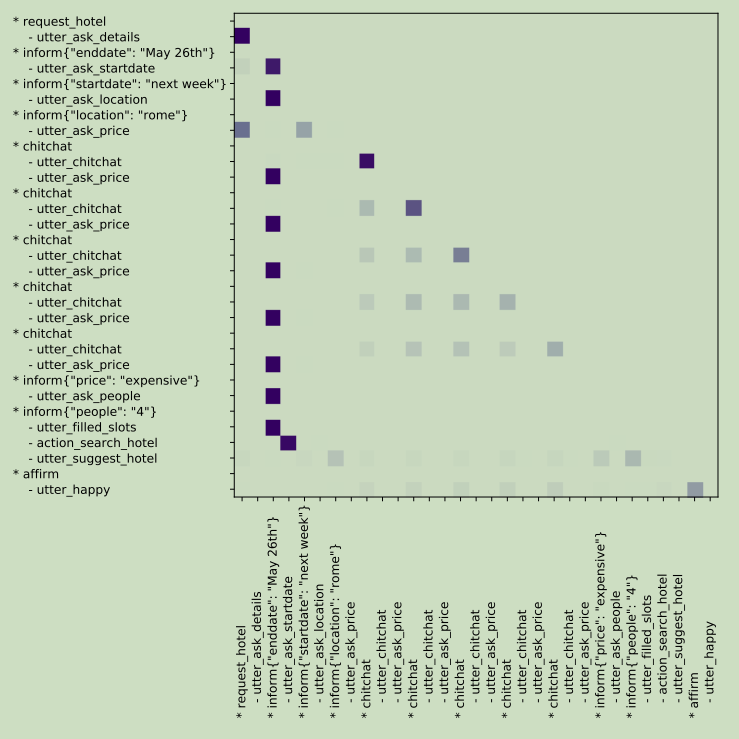### NLP Attention

Reason is the light and the light of life.

Jerry Su Mar 28, 2020 3 mins

http://jalammar.github.io/visualizing-neural-machine-translation-mechanics-of-seq2seq-models-with-attention

### Context attention - LSTM

Encoder and Decoder

``````class Attention(nn.Module):
def __init__(self, method, hidden_size):
super(Attention, self).__init__()
self.method = method
self.hidden_size = hidden_size

if self.method == 'general':
self.attention = nn.Linear(self.hidden_size, self.hidden_size)
elif self.method == 'concat':
self.attention = nn.Linear(self.hidden_size*3, self.hidden_size)
self.v = nn.Parameter(nn.init.normal_(torch.empty(self.hidden_size)))

def forward(self, hidden, encoder_outputs):
attention_energies = self.score(hidden, encoder_outputs)
attention_energies = attention_energies.t()
return F.softmax(attention_energies, dim=1).unsqueeze(1)

def score(self, hidden, encoder_output):
if self.method == 'dot':
energy = hidden.dot(encoder_output)
return energy
elif self.method == 'general':
energy = self.attention(encoder_output)
energy = hidden.dot(energy)
return energy
elif self.method == 'concat':
encoder_output = encoder_output.transpose(0, 1)
energy = self.attention(torch.cat((hidden.expand(encoder_output.size(0), -1, -1),
encoder_output), 2)).tanh()

# attention by jerry
self.attention = Attention(attn_model, hidden_size)

# Calculate attention weights from the current RNN last hidden output
attn_weights = self.attention(last_hidden.unsqueeze(0), encoder_outputs)
# Multiply attention weights to encoder outputs to get new "weighted sum" context vector
context = attn_weights.bmm(encoder_outputs)
# Concatenate weighted context vector and GRU output using Luong eq. 5
last_hidden = last_hidden.squeeze(0)
context = context.squeeze(1)
concat_input = torch.cat((last_hidden, context), 1)
concat_output = torch.tanh(self.concat(concat_input))
``````

### Self attention

Encoder or Decoder

Decoder### Self Attention Cross Attention - Transformer

``````from torch import nn, Tensor
from typing import Dict, List, Optional, Tuple

class Attention(nn.Module):
"""Multi-headed attention from 'Attention Is All You Need' paper"""

def __init__(
self,
embed_dim,
dropout=0.0,
bias=True,
encoder_decoder_attention=False,  # otherwise self_attention
):
super().__init__()
self.embed_dim = embed_dim
self.dropout = dropout

self.encoder_decoder_attention = encoder_decoder_attention
self.k_proj = nn.Linear(embed_dim, embed_dim, bias=bias)
self.v_proj = nn.Linear(embed_dim, embed_dim, bias=bias)
self.q_proj = nn.Linear(embed_dim, embed_dim, bias=bias)
self.out_proj = nn.Linear(embed_dim, embed_dim, bias=bias)
self.cache_key = "encoder_decoder" if self.encoder_decoder_attention else "self"

def _shape(self, tensor, seq_len, bsz):
"""多头注意力，隐层维度reshap
"""

def forward(
self,
query,
key: Tensor,
layer_state: Optional[Dict[str, Tensor]] = None,
output_attentions=False,
) -> Tuple[Tensor, Optional[Tensor]]:
"""Input shape: Time(SeqLen) x Batch x Channel"""
static_kv: bool = self.encoder_decoder_attention
tgt_len, bsz, embed_dim = query.size()
# get here for encoder decoder cause of static_kv
saved_state = layer_state.get(self.cache_key, {})
if "prev_key" in saved_state and static_kv:
# previous time steps are cached - no need to recompute key and value if they are static
key = None
else:
# this branch is hit by encoder
saved_state = None

# Scale q to prevent the dot product between q and k from growing too large.
q = self.q_proj(query) * self.scaling
if static_kv and key is None:  # cross-attention with cache
k = v = None
elif static_kv and key is not None:  # cross-attention no prev_key found in cache
k = self.k_proj(key)
v = self.v_proj(key)
else:  # self-attention
k = self.k_proj(query)
v = self.v_proj(query)

q = self._shape(q, tgt_len, bsz)
if k is not None:
k = self._shape(k, -1, bsz)
if v is not None:
v = self._shape(v, -1, bsz)

if saved_state:
k, v = self._concat_saved_state(k, v, saved_state, static_kv, bsz)

# Update cache
if isinstance(layer_state, dict):
cached_shape = (bsz, self.num_heads, -1, self.head_dim)  # bsz must be first for reorder_cache
layer_state[self.cache_key] = dict(prev_key=k.view(*cached_shape), prev_value=v.view(*cached_shape))

src_len = k.size(1)
# 注意力矩阵，即query与key的求出的矩阵系数
attn_weights = torch.bmm(q, k.transpose(1, 2))
assert attn_weights.size() == (bsz * self.num_heads, tgt_len, src_len)

attn_weights = attn_weights.view(bsz * self.num_heads, tgt_len, src_len)

# Note: deleted workaround to get around fork/join parallelism not supporting Optional types. on 2020/10/15

attn_weights = attn_weights.view(bsz, self.num_heads, tgt_len, src_len)
attn_weights = attn_weights.view(bsz * self.num_heads, tgt_len, src_len)
attn_weights = F.softmax(attn_weights, dim=-1)
attn_probs = F.dropout(attn_weights, p=self.dropout, training=self.training)

assert v is not None
attn_output = torch.bmm(attn_probs, v)
attn_output = attn_output.transpose(0, 1).contiguous().view(tgt_len, bsz, embed_dim)
attn_output = self.out_proj(attn_output)
if output_attentions:
attn_weights = attn_weights.view(bsz, self.num_heads, tgt_len, src_len)
else:
attn_weights = None
return attn_output, attn_weights
``````
``````
``````